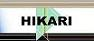| Home | Journals | Books | Paper submission | About us and our mission | News | Contact us |Site Search:

## Online Edition, Vol. 4, 2010, no. 37-40

Wei Yan
Positive solutions for fourth-order three-point boundary-value problems
Int. Journal of Math. Analysis, Vol. 4, 2010, no. 37-40, 1799-1806.

C. Selvaraj, N. Vasanthi
Coefficient bounds for certain subclasses of close-to-convex functions
Int. Journal of Math. Analysis, Vol. 4, 2010, no. 37-40, 1807-1814.

W. T. Sulaiman
A survey on Hardy-Hilbert's integral inequality
Int. Journal of Math. Analysis, Vol. 4, 2010, no. 37-40, 1815-1822.

W. T. Sulaiman
On two inequalities similar to Hardy-Hilbert's integral inequality
Int. Journal of Math. Analysis, Vol. 4, 2010, no. 37-40, 1823-1828.

W. T. Sulaiman
Some mappings in connection to Hadamard's inequality
Int. Journal of Math. Analysis, Vol. 4, 2010, no. 37-40, 1829-1836.

P. Tripathi, M. Gupta
Jungck type contractions and its application
Int. Journal of Math. Analysis, Vol. 4, 2010, no. 37-40, 1837-1850.

Hong Bin Bai, Zhi Jie Jiang, Feng Zhou
Weakly compact composition operators on Hardy spaces of the upper half-plane
Int. Journal of Math. Analysis, Vol. 4, 2010, no. 37-40, 1851-1856.

K. Raj, S. Bhougal, S. K. Sharma
Some applications of integro-substitution operators in integral equations
Int. Journal of Math. Analysis, Vol. 4, 2010, no. 37-40, 1857-1864.

Prasanna Kumar N
A generalization towards the inequalities for the polynomial and its derivative
Int. Journal of Math. Analysis, Vol. 4, 2010, no. 37-40, 1865-1871.

C. K. Tripathy, U. Mishra
An inventory model with time dependent linear deteriorating items with partial backlogging
Int. Journal of Math. Analysis, Vol. 4, 2010, no. 37-40, 1873-1879.

P.U. Chopade, K.L. Bondar, S.T. Patil, V.C. Borkar
Common fixed point theorem for some new generalized contractive mappings
Int. Journal of Math. Analysis, Vol. 4, 2010, no. 37-40, 1881-1890.

Min Chung
The construction of the Lemarie-Meyer smooth wavelets with respect to a Riesz basis
Int. Journal of Math. Analysis, Vol. 4, 2010, no. 37-40, 1891-1898.

S.M. Khursheed Haider, M. Thakur, Advin
Screen transversal lightlike submanifolds of indefinite Sasakian manifolds
Int. Journal of Math. Analysis, Vol. 4, 2010, no. 37-40, 1899-1911.

I. B. Bapna, N. Jain
Parabolic starlike and uniformly convex functions
Int. Journal of Math. Analysis, Vol. 4, 2010, no. 37-40, 1913-1921.

M. Menekse Yilmaz
On convergence of singular integral operators dependings on three parameters with radial kernels
Int. Journal of Math. Analysis, Vol. 4, 2010, no. 37-40, 1923-1928.

Nowhere Schur property in some operator spaces
Int. Journal of Math. Analysis, Vol. 4, 2010, no. 37-40, 1929-1936.

S. S. Benchalli, P. G. Patil, T. D. Rayanagoudar
On \omega-locally closed sets in bitopological spaces
Int. Journal of Math. Analysis, Vol. 4, 2010, no. 37-40, 1937-1944.

H. Chandra, B. Singh
Compactness and norm of the sum of weighted composition operators on A(D)
Int. Journal of Math. Analysis, Vol. 4, 2010, no. 37-40, 1945-1956.

C. Boonpok
Almost (g,m)-continuous functions
Int. Journal of Math. Analysis, Vol. 4, 2010, no. 37-40, 1957-1964.

C. Boonpok
On weakly (g,m)-continuous functions
Int. Journal of Math. Analysis, Vol. 4, 2010, no. 37-40, 1965-1973.

Vu Van Khuong, Tran Hong Thai
A note on global behaviuor of solutions and positive nonoscilatory solutions of rational difference equation
Int. Journal of Math. Analysis, Vol. 4, 2010, no. 37-40, 1975-1984.

Vu Van Khuong, Tran Hong Thai
Qualitative properties for a fourth-order rational difference equation (VIII)
Int. Journal of Math. Analysis, Vol. 4, 2010, no. 37-40, 1985-1993.

K. Kikina, L. Kikina
Generalized fixed point theorem in three metric spaces
Int. Journal of Math. Analysis, Vol. 4, 2010, no. 37-40, 1995-2004.